Next: Model comparison taking into Up: The Bayesian way out: Previous: Models for Galactic sources

## Relative belief updating factor for a given model

Having introduced the general ideas, let us apply them to the data of our interest. In the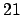-th bin in sidereal time (see Fig. 1) we haveobserved coincidences, with an expected number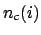due to background and an expected number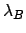(11)

due to the detectors response to g.w. signals, which depends on the model. The parameter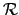is proportional to the rate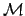of g.w. events, expressed as events/day, through the following equation: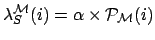(12)

where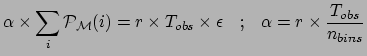is the overall efficiency of detection'',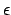the total observation time (which is 90 days),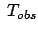the number of bins. The overall efficiency of detection is calculated from the antenna pattern as(13)

which is the ratio of the area covered by the detectors and the area which would be covered by ideal detectors (that is, having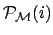=1 at all times). We have then the following likelihood for each model: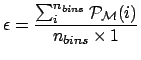(14)

with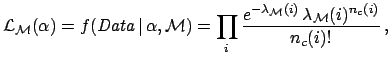(15)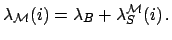is the parameter of the Poisson distribution that describes the g.w. signal given model. It can be said, in simple words, to be the number of g.w. events that could be present in each bin in a coincidence experiment having performance and exposure time as that described in Ref. . Hence,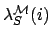(16)

gives the total number of such g.w. events. This number is the sum ofover all the bins, weighted with the pattern. Our interest is, via, to infer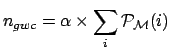and the rate. However, given the strong dependence of the inference from the priors, typical for this kind of frontier measurements, we prefer to report the result in terms of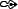functions, as discussed above.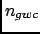The resulting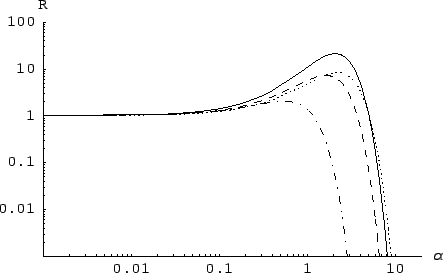's are shown in Fig. 3. Note that the figures are in log-log scale to make it clear that many orders of magnitude ofare involved.

The results are summarized in Tab. 1.

 Model GC GD GMD ISO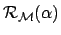4.7 6.1 4.4 12(%) 9.8 13 9.2 25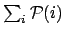21 7.2 8.2 1.9 (events)2.1 1.6 2.2 0.5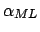1.0 0.9 1.2 0.5 E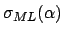2.3 1.8 2.5 0.7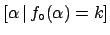1.0 0.9 1.2 0.4 (events)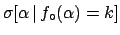10 10 10 7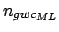5 5 5 6 E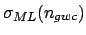11 11 11 9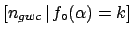5 6 5 5 (events/day)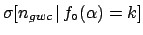1.1 0.9 1.2 0.3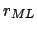0.5 0.5 0.7 0.3 E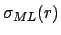1.2 1.0 1.3 0.4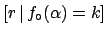0.6 0.5 0.6 0.2is the value that maximizes. The symbol ML reminds thatis the value that maximizes the likelihood (likelihood andfunction differ by a factor). Indeed, this is the result that a Maximum Likelihood (ML) analysis would produce for. The parameteris turned into g.w. rate using Eq. (12).gives how much the belief for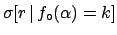increases with respect to. The higher relative belief updating factor is obtained for the Galactic Center model. Within this model, the pdf foraround 1.2 events/day gets enhanced by a factor 21 with respect to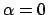.

Figure 3 shows clearly how the initial beliefs about(and therefore on) are updated, within each model. We want to stress that the final conclusion depends still on the prior beliefs. If someone thought thathad to be above 10 this person had to reconsider completely his/her beliefs, independently from the model; if another person believed that only values below 0.01 were reasonable, the experiment would not affect at all his/her beliefs, independently of the model. For this reason, the ML valuecould be misleading if erroneously associated, as it often happens, to the value around which our confidence is finally concentrated, independently from any prior knowledge. Nevertheless, and with these warnings, we report in Tab. 1 also the results obtained from a ML analysis and from a naïve Bayesian inference that assumes a uniform prior on(and therefore onand, since they differ by factors).has been evaluated from the curvature of the minus-log-likelihood around its minimum, i.e.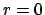. The results of the naïve Bayesian inference' are reported as expected values E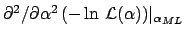and standard deviations evaluated from the final distribution. The condition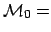has been written explicitly in Eand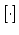, according to the Bayesian spirit. Note that, for obvious reasons, the mode of the posterior calculated using a uniform prior is exactly equivalent to the ML estimate. This observation is important to understand the slightly different results obtained with the two methods. The posterior expected value is always larger than the ML one, simply because of the asymmetry of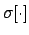.

Perhapsis the most interesting quantity to understand the conclusions of these model dependent analyzes that, we like to repeat it, do not take properly into account prior knowledge. The three physical model suggest about 10 coincidences due to g.w.'s, with a 50% uncertainty. Instead, for the unphysical model (ISO) less events are found and with larger uncertainty. Note that, for this model, the mode of the posterior (or, equivalently, the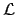estimate) gives a number of candidate events that is the difference between the total number of observed events and that expected from the background alone. Instead, for the three Galactic models, a number of events larger than this difference is attributed to the signal, as a consequence of a possibly good' time modulation recognized in the data (in other words, the method `likes to think' that, given a time distribution shape that reminds the pattern of the Galactic models, the background has most likely under-fluctuated within what is reasonably allowed by its probability distribution).

To summarize this subsection, the three Galactic models show good agreement in indicating for which values of g.w. events, or event rate, we must increase our beliefs. But the final beliefs depend on our initial ones, as explained introducing the Bayesian approach. If you think that, given your best knowledge of the models of g.w.'s sources and of g.w. interaction with cryogenic detectors, a g.w. rate on Earth of up to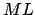event/day is quite possible, the data make you to believe that this rate is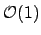event/day and that they containgenuine g.w. coincidences.Next: Model comparison taking into Up: The Bayesian way out: Previous: Models for Galactic sources
Giulio D'Agostini 2005-01-09